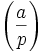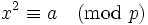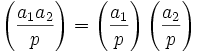# Legendre symbol

## Definition

Supposeis a prime number andis an integer. The Legendre symbol ofmodulo, denotedis defined to be:

1.ifdivides.
2.ifis a quadratic residue modulo, i.e.,does not divideandhas an integer solution for.
3.ifis a quadratic nonresidue modulo, i.e.,does not divideandhas no integer solution for.

The Legendre symbol makes sense only relative to a prime number, but it has a generalization called the Jacobi symbol which allows the modulus to be any integer. Thus, the Legendre symbol can also be defined as the restriction of the Jacobi symbol to cases where the modulus is prime.

The Legendre symbol is also a special case of a Dirichlet character modulo.

## Facts

• The Legendre symbol is multiplicative in its top portion if the prime is fixed. In other words: